# Vignette Info

This vignette is part of the R package `mipred`. It documents data analysis for the calibration of predictive models using `mipred` functions for binary outcome prediction when predictors contain missing values.

# Outlines and objectives

The package `mipred` contains two basic functions. The first is `mipred.cv`, which estimates cross-validated predictions when predictors contain missing values and using multiple imputation. The second is `mipred`, which allows users to apply the same methodology to predict outcome for novel observations, based on past calibration data. Both the new observations, as well as the calibration data may contain missing observations in the predictor data. This document describes data analysis approaches using the `mipred` package functions for the above objectives. We first discuss cross-validation of prediction with `mipred.cv`, using both the `averaging` and `rubin` methods as described in the paper by (Mertens, Banzato, and Wreede 2018) to estimate the expected prediction performance on future data. We subsequently describe use of the `mipred` function to estimated predictions on new patient data, based on past data. Finally, `mipred` package functionality and options are discussed.

``library(mipred)``

# Data preparation

The CLL data is included in the `mipred` package as a data frame. Refer to the published paper (ref) for some more detail.

``````data(cll)
head(cll)
#>   id age10     perfstat remstat           cyto          asct
#> 1  1  0.08         <NA>      CR          other no prior ASCT
#> 2  2  0.82 Karnofsky 90      PR no abnormality no prior ASCT
#> 3  3 -0.28 Karnofsky 80   SD/PD          other no prior ASCT
#> 4  4 -0.40 Karnofsky 90      PR          other no prior ASCT
#> 5  5 -0.36         <NA>   SD/PD         del11q no prior ASCT
#> 6  6  0.89 Karnofsky 90      PR           <NA>    prior ASCT
#>             donor          sex_match cond srv5y srv5y_s
#> 1 Matched Related PATfemaleDONfemale  NMA 60.02       0
#> 2      matched UD   PATfemaleDONmale  RIC 39.97       0
#> 3      matched UD PATfemaleDONfemale  NMA  8.09       1
#> 4      matched UD   PATfemaleDONmale  RIC 47.79       0
#> 5 Matched Related   PATmaleDONfemale  RIC  6.11       1
#> 6      matched UD     PATmaleDONmale  RIC 31.36       0``````

The CLL data considers a survival problem subject to (right) censoring. To get the same data as discussed in the paper for binary outcome analysis, we first apply administrative censoring and generate the one-year binary outcome as below.

``````cll_bin <- cll # Generate a new data copy
cll_bin\$srv5y_s[cll_bin\$srv5y>12] <- 0  # Apply an administrative censorship at t=12 months
cll_bin\$srv5y[cll_bin\$srv5y>12] <- 12

cll_bin\$Status[cll_bin\$srv5y_s==1] <- 1  # Define the new binary "Status" outcome variable
cll_bin\$Status[cll_bin\$srv5y_s==0] <- 0  # Encoding is 1:Dead, 0:Alive``````

Cross-tabulate the number of patients artificially censored at 12 months versus the new binary outcome status indicator.

``````binary.outcome<-cll_bin\$Status
artificially.censored<-(cll_bin\$Status==0 & cll_bin\$srv5y<12)
tableout <- table(artificially.censored,binary.outcome)
tableout
#>                      binary.outcome
#> artificially.censored   0   1
#>                 FALSE 465 184
#>                 TRUE   45   0``````

The percentage artificially censored is 100*45/694= 6.5, with 694=465+184+45 the total sample size. There are 184 events corresponding to 26.5 percent of the dataset.

Remove the original survival outcomes from the data frame before proceeding.

``````cll_bin\$srv5y <- NULL # Remove the original censoring and follow-up time
cll_bin\$srv5y_s <- NULL``````

This completely defines the data.frame for use in further analysis. Aside from the (binary) outcome variable (`Status`), we have a single continuous predictor (`age10`). All other predictor variables (7 in total) are categorical, which gives 8 predictors in total. The first column contains a numeric identification variable with unique integers (`1:694`) for each individual.

# Data exploration

It makes sense to first check the missing data pattern as well as some simple exploratory data summaries on predictors. Load the mice package if not already loaded.

``````library(mice)
md.pattern(cll_bin, rotate.names = TRUE)``````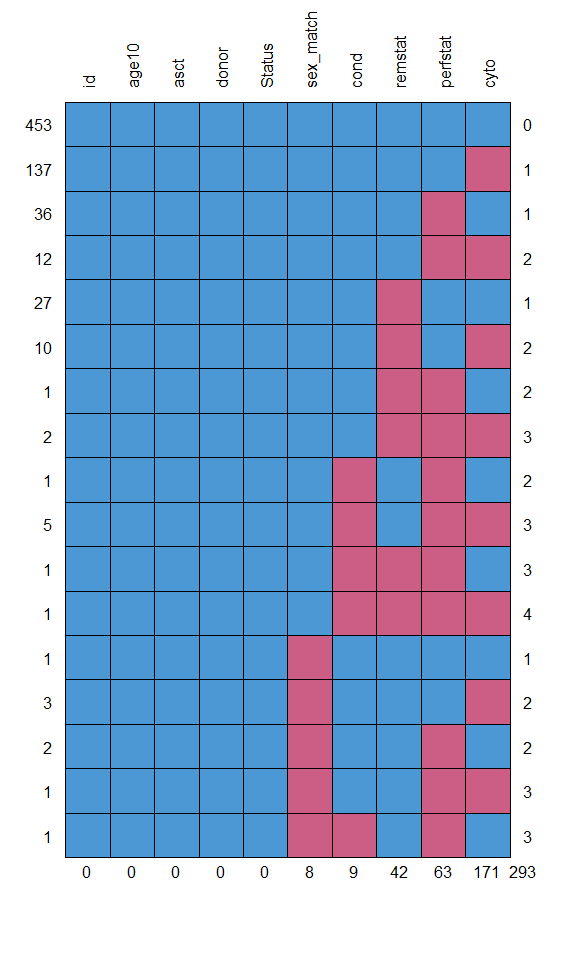``````#>     id age10 asct donor Status sex_match cond remstat perfstat cyto
#> 453  1     1    1     1      1         1    1       1        1    1   0
#> 137  1     1    1     1      1         1    1       1        1    0   1
#> 36   1     1    1     1      1         1    1       1        0    1   1
#> 12   1     1    1     1      1         1    1       1        0    0   2
#> 27   1     1    1     1      1         1    1       0        1    1   1
#> 10   1     1    1     1      1         1    1       0        1    0   2
#> 1    1     1    1     1      1         1    1       0        0    1   2
#> 2    1     1    1     1      1         1    1       0        0    0   3
#> 1    1     1    1     1      1         1    0       1        0    1   2
#> 5    1     1    1     1      1         1    0       1        0    0   3
#> 1    1     1    1     1      1         1    0       0        0    1   3
#> 1    1     1    1     1      1         1    0       0        0    0   4
#> 1    1     1    1     1      1         0    1       1        1    1   1
#> 3    1     1    1     1      1         0    1       1        1    0   2
#> 2    1     1    1     1      1         0    1       1        0    1   2
#> 1    1     1    1     1      1         0    1       1        0    0   3
#> 1    1     1    1     1      1         0    0       1        0    1   3
#>      0     0    0     0      0         8    9      42       63  171 293``````

There are 293 missing values in total. There are 241=694-453 records containing missing values. Most missing values occur in variables `cyto` (171), `perfstat` (63) and `remstat` (42). Age is the only continuous predictor. Since we wish to investigate predictions and (cross-)validation in particular, it makes sense to explore the distribution of factor levels for the categorical predictors.

``````table(cll_bin\$cyto)
#>
#>         del17p         del11q          other no abnormality
#>            144            142            166             71
table(cll_bin\$perfstat)
#>
#>  Karnofsky 100   Karnofsky 90   Karnofsky 80 Karnofsky <=70
#>            186            307            113             25
table(cll_bin\$remstat)
#>
#>    CR    PR SD/PD
#>    84   344   224
table(cll_bin\$asct)
#>
#> no prior ASCT    prior ASCT
#>           620            74
table(cll_bin\$donor)
#>
#>         Matched Related              matched UD partially mismatched UD
#>                     283                     327                      84
table(cll_bin\$sex_match)
#>
#>     PATmaleDONmale   PATmaleDONfemale   PATfemaleDONmale
#>                363                145                 98
#> PATfemaleDONfemale
#>                 80
table(cll_bin\$cond)
#>
#> NMA RIC MAC
#> 223 360 102``````

These tables by default exclude the missing values. Note how the lowest factor level of remstat (Karnofsky <=70) is very sparse with only 25 observations. Similar output which retains the information on missing values can also be obtained from

``````summary(cll_bin)
#>       id                age10                    perfstat    remstat
#>  Length:694         Min.   :-3.58000   Karnofsky 100 :186   CR   : 84
#>  Class :character   1st Qu.:-0.58750   Karnofsky 90  :307   PR   :344
#>  Mode  :character   Median : 0.04000   Karnofsky 80  :113   SD/PD:224
#>                     Mean   :-0.06703   Karnofsky <=70: 25   NA's : 42
#>                     3rd Qu.: 0.51000   NA's          : 63
#>                     Max.   : 1.91000
#>              cyto                asct                         donor
#>  del17p        :144   no prior ASCT:620   Matched Related        :283
#>  del11q        :142   prior ASCT   : 74   matched UD             :327
#>  other         :166                       partially mismatched UD: 84
#>  no abnormality: 71
#>  NA's          :171
#>
#>               sex_match     cond         Status
#>  PATmaleDONmale    :363   NMA :223   Min.   :0.0000
#>  PATmaleDONfemale  :145   RIC :360   1st Qu.:0.0000
#>  PATfemaleDONmale  : 98   MAC :102   Median :0.0000
#>  PATfemaleDONfemale: 80   NA's:  9   Mean   :0.2651
#>  NA's              :  8              3rd Qu.:1.0000
#>                                      Max.   :1.0000``````

# Model assessment via internal validation

## Estimate predictive performance using mipred.cv functions

We first estimate prediction results using internal validation on the cll_bin data, using all predictors and running 10-fold cross-validation (argument `folds`) with 10 imputations (argument `nimp`) for each prediction. Note the `-1` notation in `cll_bin[,-1]` is to remove the first column containing the observation identification number.

``````predcv_av <-
mipred.cv(
Status ~ age10 + perfstat + remstat + cyto + asct + donor + sex_match + cond,
family = binomial,
data = cll_bin[, -1],
nimp = 10,
folds = 10
)``````

The above code implements cross-validation for the averaging approach as described in the paper by Mertens, Banzato, and Wreede (2018). In brief, to generate 10 imputations, 10 copies of the data matrix are made and a separate (in this case 10-fold) fold-partition is defined for each matrix. In each matrix copy, each fold is then considered in turn as a validation set (with the remainder of the data as calibration) and the outcomes are removed (artificially set to missing) in the left-out fold. A single imputation is then generated on this data matrix. The (imputed) training potion of the data is then used to fit a (logistic regression) model, which is applied to the imputed data in the left-out (training) fold. This process is repeated for all folds. By applying the above calculation to all matrices, we obtain 10 cross-validated predictions, each on a single imputation (10 in total), for each observation.

The returned object is a list containing three elements: the function `call`, as well as the predictions on the response scale (fitted probabilities in this case, in matrix `pred`) and the linear predictor (`linpred`). The prediction method used is the averaging method by default. The two prediction matrices are each saved in a matrix with 694 rows by 10 columns, with each column containing prediction results based on the prediction model calibrated on a single imputation. Rows correspond to the observations in the data matrix, in same order. The cross-validation fold-definitions used are different from column to column in the averaging approach. The function linking both matrices `pred`and `linpred` is the logit link (by default of the family specification `family = binomial`).

``````head(predcv_av\$pred)
#>        [,1]      [,2]      [,3]      [,4]       [,5]      [,6]      [,7]
#> 1 0.1128680 0.1269674 0.1167276 0.1071034 0.09534024 0.1364446 0.1249438
#> 2 0.1842251 0.3527772 0.2507300 0.2949510 0.34232462 0.3103954 0.2619670
#> 3 0.2664314 0.2036986 0.2538854 0.2654940 0.25080963 0.2997654 0.2550819
#> 4 0.1787659 0.2079587 0.1481280 0.1637103 0.18579302 0.1472017 0.1553044
#> 5 0.3385423 0.3818292 0.3271067 0.5398961 0.37388643 0.4239001 0.3289980
#> 6 0.4020641 0.3119868 0.3349398 0.3885224 0.38323241 0.3616578 0.3441534
#>        [,8]       [,9]     [,10]
#> 1 0.1343942 0.09427873 0.1635920
#> 2 0.2080130 0.27142416 0.3150706
#> 3 0.2654622 0.27826623 0.4013794
#> 4 0.1438300 0.18015161 0.1738653
#> 5 0.2840912 0.45497986 0.4279299
#> 6 0.3767491 0.37957767 0.2956087``````
``````head(predcv_av\$linpred)
#>         [,1]       [,2]       [,3]       [,4]       [,5]       [,6]
#> 1 -2.0617743 -1.9280424 -2.0237904 -2.1206760 -2.2501070 -1.8451392
#> 2 -1.4879802 -0.6068539 -1.0947229 -0.8714580 -0.6529520 -0.7982714
#> 3 -1.0128045 -1.3633364 -1.0779962 -1.0176060 -1.0942989 -0.8484150
#> 4 -1.5247311 -1.3372738 -1.7493596 -1.6308770 -1.4775813 -1.7567195
#> 5 -0.6697969 -0.4817915 -0.7213004  0.1599245 -0.5155797 -0.3067832
#> 6 -0.3968719 -0.7908471 -0.6859269 -0.4535274 -0.4758507 -0.5681759
#>         [,7]       [,8]       [,9]      [,10]
#> 1 -1.9464241 -1.8626526 -2.2624760 -1.6317413
#> 2 -1.0357701 -1.3369445 -0.9874089 -0.7765190
#> 3 -1.0716894 -1.0177692 -0.9530780 -0.3997211
#> 4 -1.6935891 -1.7838370 -1.5153206 -1.5584771
#> 5 -0.7127206 -0.9242577 -0.1805696 -0.2903023
#> 6 -0.6448395 -0.5033696 -0.4913412 -0.8682974``````

To obtain predictions calculated from pooled Rubin’s rules models, we need to set the method option explicitly to “rubin”.

``````predcv_rb <-
mipred.cv(
Status ~ age10 + perfstat + remstat + cyto + asct + donor + sex_match + cond,
family = binomial,
data = cll_bin[, -1],
nimp = 10,
folds = 10,
method = "rubin"
)``````

For both the “rubin” and “averaging” method, the above codes generate 10 predictions (1 for each imputation, of which we have 10 in total). With the averaging method, these will typically differ, because a separate prediction model is generated for any left-out datum based on each single-imputed dataset. The “rubin” approach uses the pooled model as single prediction model. Nevertheless, any record to be predicted which itself contains missing data will also be imputed. Thus, there will generally also be 10 distinct predictions for such observations with the (pooled) rubin approach. Records which are fully observed will have the same predictions across all imputations as we are applying the same - pooled - model on the same fully observed record of predictors.

``````head(predcv_rb\$pred)
#>        [,1]      [,2]      [,3]      [,4]      [,5]      [,6]      [,7]
#> 1 0.1128963 0.1016203 0.1016203 0.1016203 0.1128963 0.1128963 0.1128963
#> 2 0.2723166 0.2723166 0.2723166 0.2723166 0.2723166 0.2723166 0.2723166
#> 3 0.2329543 0.2329543 0.2329543 0.2329543 0.2329543 0.2329543 0.2329543
#> 4 0.1825556 0.1825556 0.1825556 0.1825556 0.1825556 0.1825556 0.1825556
#> 5 0.3234484 0.4475113 0.4475113 0.3160147 0.3234484 0.3160147 0.3234484
#> 6 0.3282684 0.3255040 0.3282684 0.3077205 0.3255040 0.4103372 0.3255040
#>        [,8]      [,9]     [,10]
#> 1 0.1016203 0.1016203 0.1016203
#> 2 0.2723166 0.2723166 0.2723166
#> 3 0.2329543 0.2329543 0.2329543
#> 4 0.1825556 0.1825556 0.1825556
#> 5 0.3234484 0.3234484 0.4475113
#> 6 0.3255040 0.3282684 0.4103372``````
``````head(predcv_rb\$linpred)
#>         [,1]       [,2]       [,3]       [,4]       [,5]       [,6]
#> 1 -2.0614922 -2.1793497 -2.1793497 -2.1793497 -2.0614922 -2.0614922
#> 2 -0.9829007 -0.9829007 -0.9829007 -0.9829007 -0.9829007 -0.9829007
#> 3 -1.1917041 -1.1917041 -1.1917041 -1.1917041 -1.1917041 -1.1917041
#> 4 -1.4991283 -1.4991283 -1.4991283 -1.4991283 -1.4991283 -1.4991283
#> 5 -0.7379689 -0.2107314 -0.2107314 -0.7721476 -0.7379689 -0.7721476
#> 6 -0.7160274 -0.7285910 -0.7160274 -0.8107981 -0.7285910 -0.3625714
#>         [,7]       [,8]       [,9]      [,10]
#> 1 -2.0614922 -2.1793497 -2.1793497 -2.1793497
#> 2 -0.9829007 -0.9829007 -0.9829007 -0.9829007
#> 3 -1.1917041 -1.1917041 -1.1917041 -1.1917041
#> 4 -1.4991283 -1.4991283 -1.4991283 -1.4991283
#> 5 -0.7379689 -0.7379689 -0.7379689 -0.2107314
#> 6 -0.7285910 -0.7285910 -0.7160274 -0.3625714``````

Note how in the above prediction matrices `pred` and `linpred` the entries in rows 2-4 are the same for all 10 predictions, because these prediction are calculated from the same pooled model on observations which do not contain missing values. Observations 1, 5 and 6 however do contain missing values and hence, the imputations for these missing data also change across the 10 predictions, even though the prediction model itself is fixed. The final prediction for any record can be obtained by taking averages, medians or other suitable statistic across the distinct predictions. Here we use means for example, but other applications may well require another combination.

``````predfinal_av <- apply(predcv_av\$pred,1,mean)
predfinal_rb <- apply(predcv_rb\$pred,1,mean)``````

We can investigate some plots to get a feel for the calibrated predictions. Before proceeding, we first generate a missingness indicator for each observation, to distinguish predictions on fully observed records from those on records containing missing values, in tables and figures.

``````missrownrs<-sort(unique (unlist (lapply (cll_bin, function (x) which (is.na (x))))))
miss  <- matrix(0, nrow=nrow(cll_bin), ncol=1)
miss[missrownrs]<-1``````

The below figure plots the combined predictions versus the status outcome and also compares predictions between the averaging and rubin combination method. Plotted predictions are colored red for observations containing missing predictor data and black otherwise. In the second row of plots, the left-most figure plots predictions from the rubin method versus those from the averaging approach. The second right-most figure, plots differences between predictions from both approaches versus the averaged predictions obtained from both the rubin and averaging method.

``````par(mfrow=c(2,2),pty="s")
boxplot(predfinal_av~cll_bin\$Status)
title("averaging method")
boxplot(predfinal_rb~cll_bin\$Status)
title("rubin method")
matplot(predfinal_av,predfinal_rb,pch=19,type="n",xlab="averaging method",ylab="rubin method")
matpoints(predfinal_av[miss==1],predfinal_rb[miss==1],col=2,pch=1)
matpoints(predfinal_av[miss==0],predfinal_rb[miss==0],col=1,pch=1)
title("rubin versus averaging method")
matplot((predfinal_av+predfinal_rb)/2,predfinal_av-predfinal_rb,pch=19,type="n",
xlab="average prediction",ylab="prediction difference")
matpoints((predfinal_av[miss==1]+predfinal_rb[miss==1])/2,
predfinal_av[miss==1]-predfinal_rb[miss==1],col=2,pch=1)
matpoints((predfinal_av[miss==0]+predfinal_rb[miss==0])/2,
predfinal_av[miss==0]-predfinal_rb[miss==0],col=1,pch=1)
title("differences versus average prediction")``````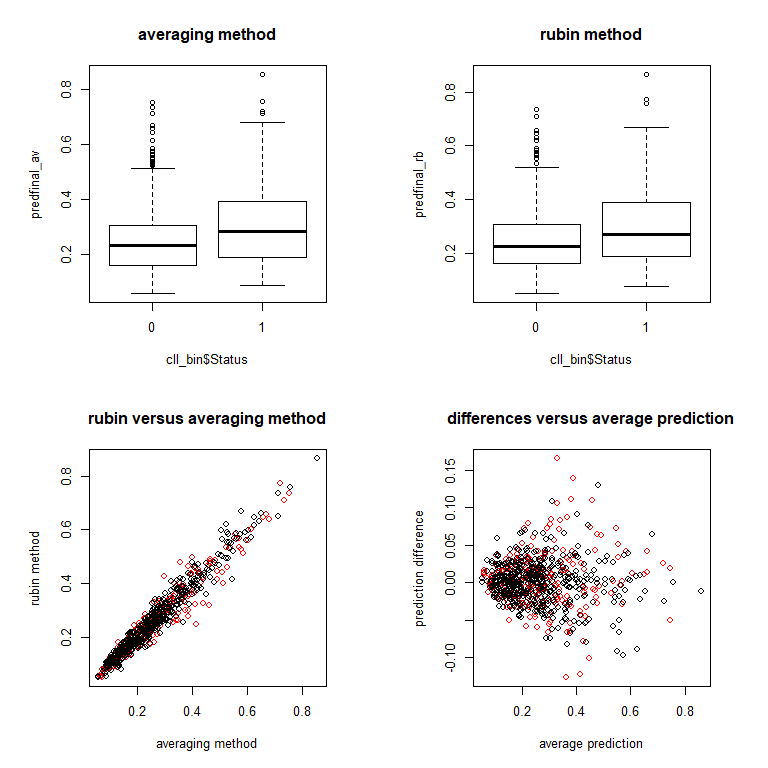The combined predictions as obtained from the mean seem to be similar between both approaches, but some variation is observed between predictions from both methods. Most of this variation is due to between-imputation variation. This raises some obvious questions.

1. The first is how large is the variation of predictions associated with any specific calibration approach (either averaging or rubin)?
2. Secondly, is variation different between both methodologies?
3. A logical follow-up question would be to what extent such variation can be reduced by increasing numbers of imputations.
4. Finally, we could try to estimate the number of imputations that would be sufficient to reduce the variation to acceptable levels.

We can investigate the impact of increased numbers of imputations for each prediction combination approach by running multiple replicates of the above analysis and with different numbers of imputations. Note the below code can take considerable time to run.

``````repslist_av <- vector("list", 3)
m <- c(1, 10, 100)
for (counter in 1:3) {
reps_av <- array(NA, dim = c(nrow(cll_bin), m[counter], 10))
for (rep in 1:10) {
reps_av[, , rep] <-
mipred.cv(
Status ~ age10 + perfstat + remstat + cyto + asct + donor + sex_match + cond,
family = binomial,
data = cll_bin[,-1],
nimp = m[counter],
folds = 10
)[]
}
repslist_av[[counter]] <- reps_av
}``````

Now generate same analysis for the “rubin” method.

``````repslist_rb <- vector("list", 3)
m <- c(1, 10, 100)
for (counter in 1:3) {
reps_rb <- array(NA, dim = c(nrow(cll_bin), m[counter], 10))
for (rep in 1:10) {
reps_rb[, , rep] <-
mipred.cv(
Status ~ age10 + perfstat + remstat + cyto + asct + donor + sex_match + cond,
family = binomial,
data = cll_bin[,-1],
nimp = m[counter],
folds = 10,
method = "rubin"
)[]
}
repslist_rb[[counter]] <- reps_rb
}``````

## Summarize results using plots

We first investigate the averaging method. To investigate the spread of individual predictions, calculated from models on a single imputation, we first plot the these constituent (individual) predictions (from repslist_av) versus the combined predictions (the means) for the averaging method. Use distinct plotting symbols for predictions based on fully observed records versus records that contained missing values. Use only the first replicate from `nimp=100` (the third component of `repslist_av`, corresponding to results for 100 imputations. Calculate the final prediction for each patient for this replicate by combining the individual predictions using means.

``avcv_mean3 <- apply(repslist_av[][,,1],1,mean) # one hundred imputations ``

Now make the plot.

``````par(mfrow=c(1,2),pty="s")
matplot(avcv_mean3,repslist_av[][,,1],pch=19,type="n",
xlab="ordered mean probability",ylab="individual imputed predictions")
matpoints(avcv_mean3[miss==1],repslist_av[][miss==1,,1],col=2,pch=1)
matpoints(avcv_mean3[miss==0],repslist_av[][miss==0,,1],col=1,pch=1)

matplot(avcv_mean3,
repslist_av[][,,1]-avcv_mean3%*%matrix(1,nrow=1,ncol=ncol(repslist_av[][,,1])),
pch=19,type="n",xlab="ordered mean probability",ylab="mean-centered individual imputed predictions")
matpoints(avcv_mean3[miss==1],
repslist_av[][miss==1,,1]-
avcv_mean3[miss==1]%*%matrix(1,nrow=1,ncol=ncol(repslist_av[][,,1])),
col=2,pch=1)
matpoints(avcv_mean3[miss==0],
repslist_av[][miss==0,,1]-
avcv_mean3[miss==0]%*%matrix(1,nrow=1,ncol=ncol(repslist_av[][,,1])),
col=1,pch=1)``````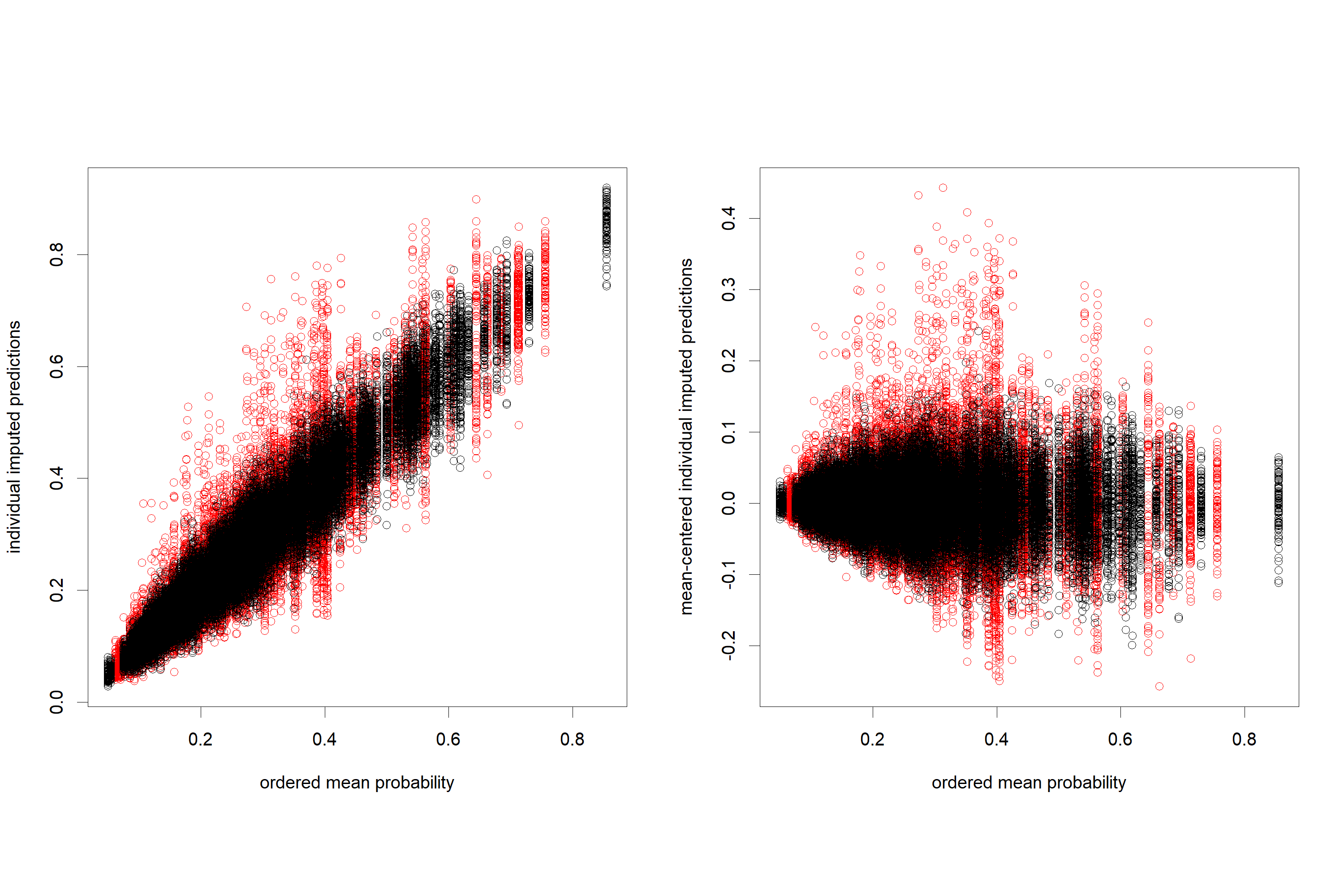The left plot shows individual predictions (from single-imputation-based models) versus the mean prediction. The right plot shows mean-centered predictions versus mean prediction. As can be seen, the individual predictions have very high levels of variation (around the mean prediction). Predictions on (partially missing records - plotted red) are much more variable than those on fully-observed records. Variation tends to decrease as the mean prediction approaches either 0 or 1.

Now investigate the Rubin method results in the same manner. Again only consider a single replication for `nimp=100`. Plot the constituent (individual) predictions (from repslist_rb) versus the combined predictions (the means) for the rubin method. Use distinct plotting symbols for predictions based on fully observed records versus records that contained missing values.

First, calculate the final prediction for each patient for this replicate by combining the individual predictions using means.

``rbcv_mean3 <- apply(repslist_rb[][,,1],1,mean)``

Now make the plots.

``````par(mfrow=c(1,2),pty="s")
matplot(rbcv_mean3,repslist_rb[][,,1],pch=19,type="n",
xlab="ordered mean probability",ylab="individual imputed predictions")
matpoints(rbcv_mean3[miss==1],repslist_rb[][miss==1,,1],col=2,pch=1)
matpoints(rbcv_mean3[miss==0],repslist_rb[][miss==0,,1],col=1,pch=1)

matplot(rbcv_mean3,
repslist_rb[][,,1]-rbcv_mean3%*%matrix(1,nrow=1,ncol=ncol(repslist_rb[][,,1])),
pch=19,type="n",
xlab="ordered mean probability",ylab="mean-centered individual imputed predictions")
matpoints(rbcv_mean3[miss==1],
repslist_rb[][miss==1,,1]-
rbcv_mean3[miss==1]%*%matrix(1,nrow=1,ncol=ncol(repslist_rb[][,,1])),
col=2,pch=1)
matpoints(rbcv_mean3[miss==0],
repslist_rb[][miss==0,,1]-
rbcv_mean3[miss==0]%*%matrix(1,nrow=1,ncol=ncol(repslist_rb[][,,1])),
col=1,pch=1)``````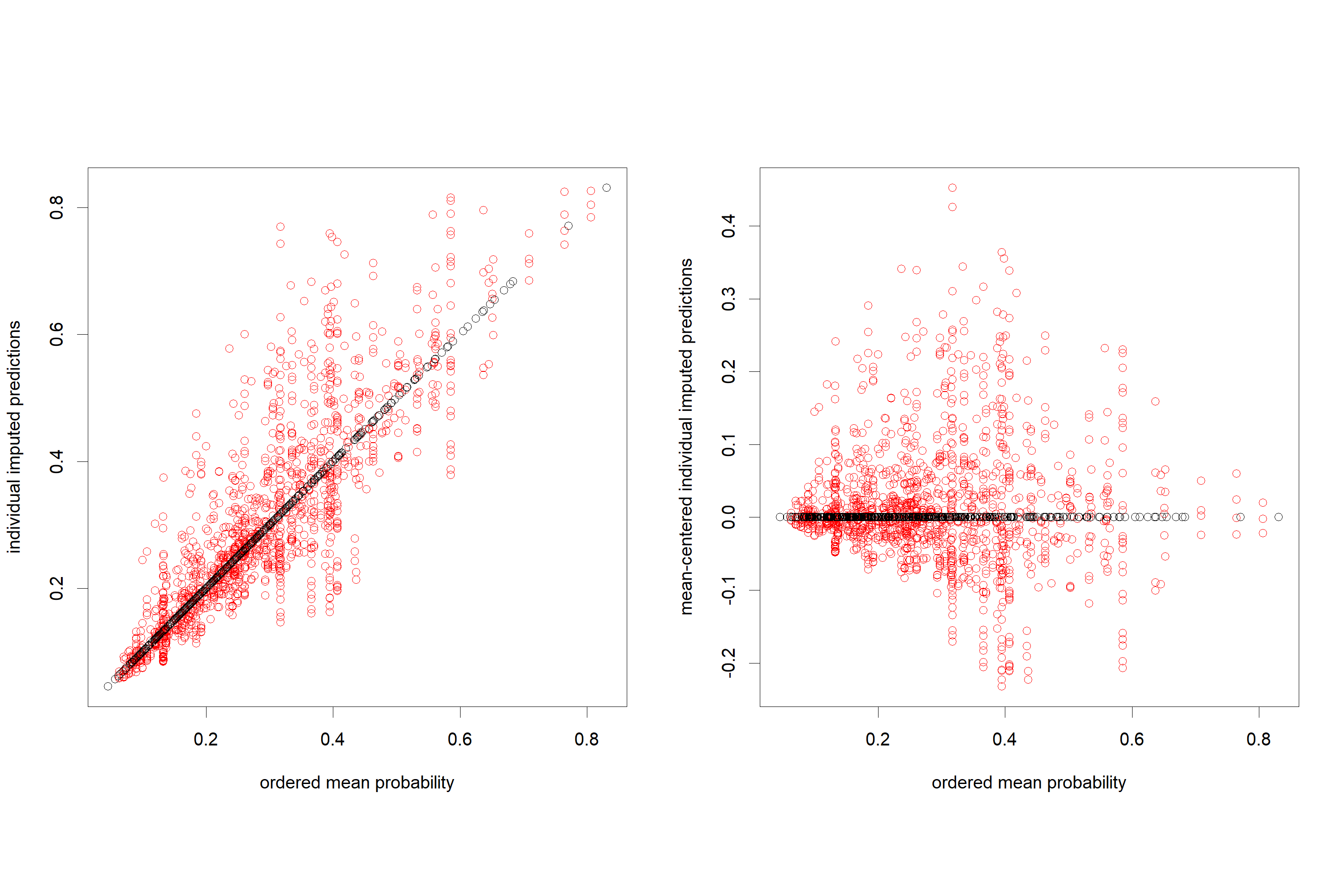The left plot shows individual predictions (from the Rubin-rule pooled model on each of the [possibly imputed] records) versus the mean prediction. The right plot shows mean-centered predictions versus mean prediction. As can be seen, the individual predictions have very high levels of variation (around the mean level) when predicting on records which are partially observed (contain missing values). Note how there is no variability in predictions on fully-observed records now between the imputations, because we are predicting from a single pooled model. Predictions on (partially missing records - plotted red) are however variable because we also need to impute the missing data each time we want to predict (so there are `nimp=100` distinct predictions in that case). Variation tends to decrease as the mean prediction approaches either 0 or 1.

Next we study the differences (variation) between the predictions obtained from the averaging method when generating replicates of the prediction, calculated from the same number of imputations, but with distinct imputations. We repeat this analysis for `nimp=1`, `nimp=10` and `nimp=100` imputations. In other words, we replicate the prediction analysis and investigate the impact of increasing the number of imputations used on the stability of the prediction.

In the below plots, the top row of plots show the replicated predictions versus the mean prediction. The bottom row of plots displays the mean-centered replicate predictions versus mean prediction. First calculate the mean across all imputations and replications.

``````avcv_means1 <- apply(repslist_av[],1,mean) # one imputation
avcv_means2 <- apply(repslist_av[],1,mean) # ten imputations
avcv_means3 <- apply(repslist_av[],1,mean) # one hundred imputations ``````

Now make the plots.

``````par(mfrow=c(2,3),pty="s")
matplot(avcv_means1,apply(repslist_av[],c(1,3),mean),pch=19,type="n",
xlab="ordered mean probability",ylab="individual imputed predictions")
matpoints(avcv_means1[miss==1],apply(repslist_av[][miss==1,,,drop=FALSE],c(1,3),mean),
col=2,pch=1)
matpoints(avcv_means1[miss==0],apply(repslist_av[][miss==0,,,drop=FALSE],c(1,3),mean),
col=1,pch=1)
title('single imputation')

matplot(avcv_means2,apply(repslist_av[],c(1,3),mean),pch=19,type="n",
xlab="ordered mean probability",ylab="individual imputed predictions")
matpoints(avcv_means2[miss==1],apply(repslist_av[][miss==1,,],c(1,3),mean),
col=2,pch=1)
matpoints(avcv_means2[miss==0],apply(repslist_av[][miss==0,,],c(1,3),mean),
col=1,pch=1)
title('10 imputations')

matplot(avcv_means3,apply(repslist_av[],c(1,3),mean),pch=19,type="n",
xlab="ordered mean probability",ylab="individual imputed predictions")
matpoints(avcv_means3[miss==1],apply(repslist_av[][miss==1,,],c(1,3),mean),
col=2,pch=1)
matpoints(avcv_means3[miss==0],apply(repslist_av[][miss==0,,],c(1,3),mean),col=1,pch=1)
title('100 imputations')

matplot(avcv_means1,apply(repslist_av[],c(1,3),mean)-avcv_means1%*%matrix(1,nrow=1,ncol=10),
pch=19,type="n",xlab="ordered mean probability",ylab="individual imputed predictions")
matpoints(avcv_means1[miss==1],
apply(repslist_av[][miss==1,,,drop=FALSE],c(1,3),mean)-
avcv_means1[miss==1]%*%matrix(1,nrow=1,ncol=10),
col=2,pch=1)
matpoints(avcv_means1[miss==0],
apply(repslist_av[][miss==0,,,drop=FALSE],c(1,3),mean)-
avcv_means1[miss==0]%*%matrix(1,nrow=1,ncol=10),
col=1,pch=1)

matplot(avcv_means2,
apply(repslist_av[],c(1,3),mean)-avcv_means2%*%matrix(1,nrow=1,ncol=10),
pch=19,type="n",xlab="ordered mean probability",ylab="individual imputed predictions")
matpoints(avcv_means2[miss==1],
apply(repslist_av[][miss==1,,],c(1,3),mean)-
avcv_means2[miss==1]%*%matrix(1,nrow=1,ncol=10),
col=2,pch=1)
matpoints(avcv_means2[miss==0],
apply(repslist_av[][miss==0,,],c(1,3),mean)-
avcv_means2[miss==0]%*%matrix(1,nrow=1,ncol=10),
col=1,pch=1)

matplot(avcv_means3,
apply(repslist_av[],c(1,3),mean)-avcv_means3%*%matrix(1,nrow=1,ncol=10),
pch=19,type="n",xlab="ordered mean probability",ylab="individual imputed predictions")
matpoints(avcv_means3[miss==1],
apply(repslist_av[][miss==1,,],c(1,3),mean)-
avcv_means3[miss==1]%*%matrix(1,nrow=1,ncol=10),
col=2,pch=1)
matpoints(avcv_means3[miss==0],
apply(repslist_av[][miss==0,,],c(1,3),mean)-
avcv_means3[miss==0]%*%matrix(1,nrow=1,ncol=10),
col=1,pch=1)``````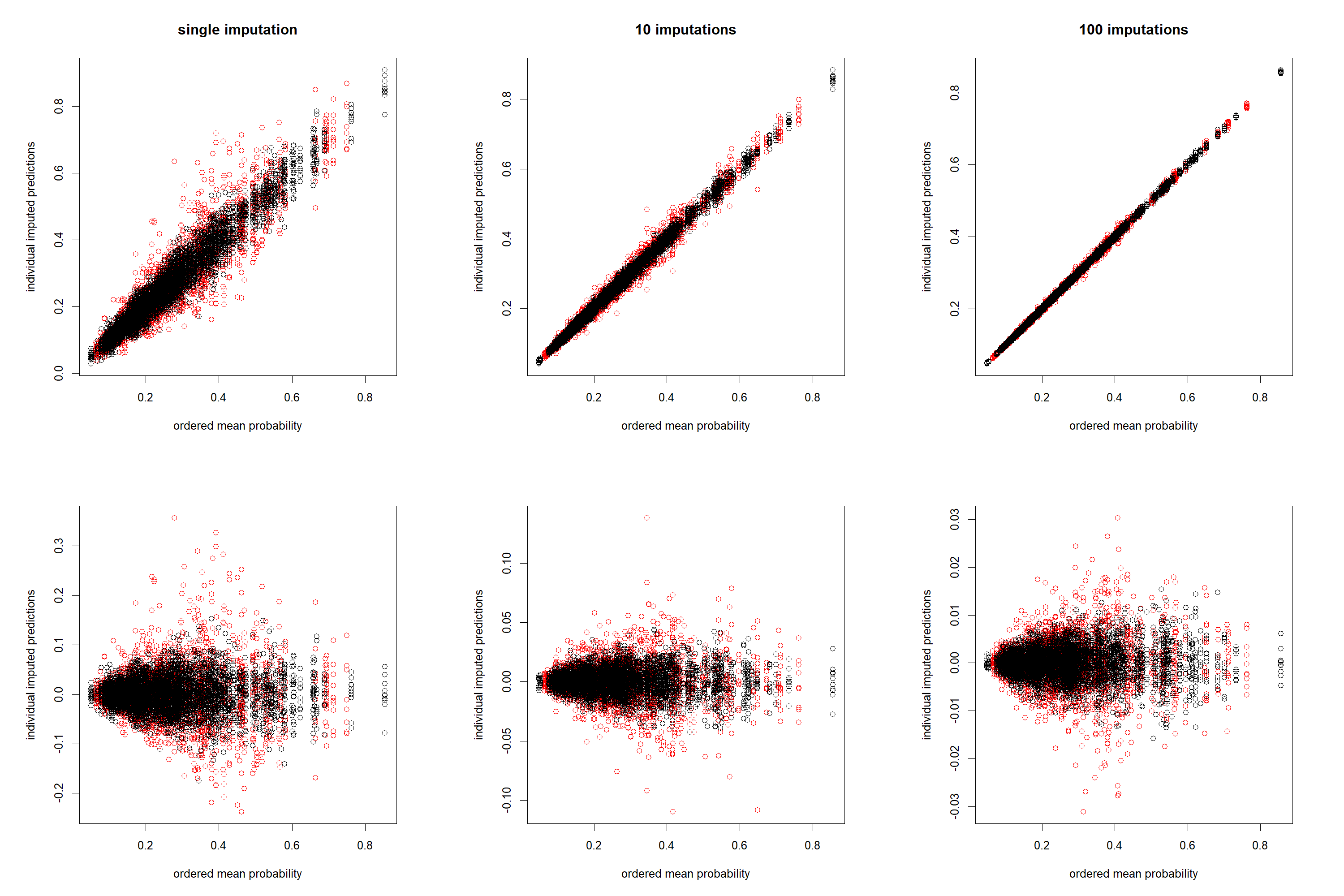We observe prediction on partially observed records to have lower levels of (between-replication) variation as compared to prediction on fully observed data. Variation reduces substantially when the numbers of imputations used are increased. Observation with mean predicted probabilities close to either 0 or 1 have lower between-replication variation.

Next investigate the dispersal between the predictions for replicates of the analyses with different number of imputations used, for the rubin method. Top row of plots is replicate predictions versus the mean prediction. Bottom row of plot is mean-centered replicate predictions versus mean prediction. First calculate the mean across all imputations and replications.

``````rbcv_means1 <- apply(repslist_rb[],1,mean)
rbcv_means2 <- apply(repslist_rb[],1,mean)
rbcv_means3 <- apply(repslist_rb[],1,mean)``````

Now make the plots.

``````par(mfrow=c(2,3),pty="s")
matplot(rbcv_means1,apply(repslist_rb[],c(1,3),mean),
pch=19,type="n",xlab="ordered mean probability",ylab="individual imputed predictions")
matpoints(rbcv_means1[miss==1],apply(repslist_rb[][miss==1,,,drop=FALSE],c(1,3),mean),
col=2,pch=1)
matpoints(rbcv_means1[miss==0],apply(repslist_rb[][miss==0,,,drop=FALSE],c(1,3),mean),
col=1,pch=1)
title('single imputation')

matplot(rbcv_means2,apply(repslist_rb[],c(1,3),mean),
pch=19,type="n",xlab="ordered mean probability",ylab="individual imputed predictions")
matpoints(rbcv_means2[miss==1],apply(repslist_rb[][miss==1,,],c(1,3),mean),col=2,pch=1)
matpoints(rbcv_means2[miss==0],apply(repslist_rb[][miss==0,,],c(1,3),mean),col=1,pch=1)
title('10 imputations')

matplot(rbcv_means3,apply(repslist_rb[],c(1,3),mean),
pch=19,type="n",xlab="ordered mean probability",ylab="individual imputed predictions")
matpoints(rbcv_means3[miss==1],apply(repslist_rb[][miss==1,,],c(1,3),mean),col=2,pch=1)
matpoints(rbcv_means3[miss==0],apply(repslist_rb[][miss==0,,],c(1,3),mean),col=1,pch=1)
title('100 imputations')

matplot(rbcv_means1,
apply(repslist_rb[],c(1,3),mean)-rbcv_means1%*%matrix(1,nrow=1,ncol=10),
pch=19,type="n",xlab="ordered mean probability",ylab="individual imputed predictions")
matpoints(rbcv_means1[miss==1],
apply(repslist_rb[][miss==1,,,drop=FALSE],c(1,3),mean)-
rbcv_means1[miss==1]%*%matrix(1,nrow=1,ncol=10),
col=2,pch=1)
matpoints(rbcv_means1[miss==0],
apply(repslist_rb[][miss==0,,,drop=FALSE],c(1,3),mean)-
rbcv_means1[miss==0]%*%matrix(1,nrow=1,ncol=10),
col=1,pch=1)

matplot(rbcv_means2,
apply(repslist_rb[],c(1,3),mean)-rbcv_means2%*%matrix(1,nrow=1,ncol=10),
pch=19,type="n",xlab="ordered mean probability",ylab="individual imputed predictions")
matpoints(rbcv_means2[miss==1],
apply(repslist_rb[][miss==1,,],c(1,3),mean)-
rbcv_means2[miss==1]%*%matrix(1,nrow=1,ncol=10),
col=2,pch=1)
matpoints(rbcv_means2[miss==0],
apply(repslist_rb[][miss==0,,],c(1,3),mean)-
rbcv_means2[miss==0]%*%matrix(1,nrow=1,ncol=10),
col=1,pch=1)

matplot(rbcv_means3,
apply(repslist_rb[],c(1,3),mean)-rbcv_means3%*%matrix(1,nrow=1,ncol=10),
pch=19,type="n",xlab="ordered mean probability",ylab="individual imputed predictions")
matpoints(rbcv_means3[miss==1],
apply(repslist_rb[][miss==1,,],c(1,3),mean)-
rbcv_means3[miss==1]%*%matrix(1,nrow=1,ncol=10),
col=2,pch=1)
matpoints(rbcv_means3[miss==0],
apply(repslist_rb[][miss==0,,],c(1,3),mean)-
rbcv_means3[miss==0]%*%matrix(1,nrow=1,ncol=10),
col=1,pch=1)``````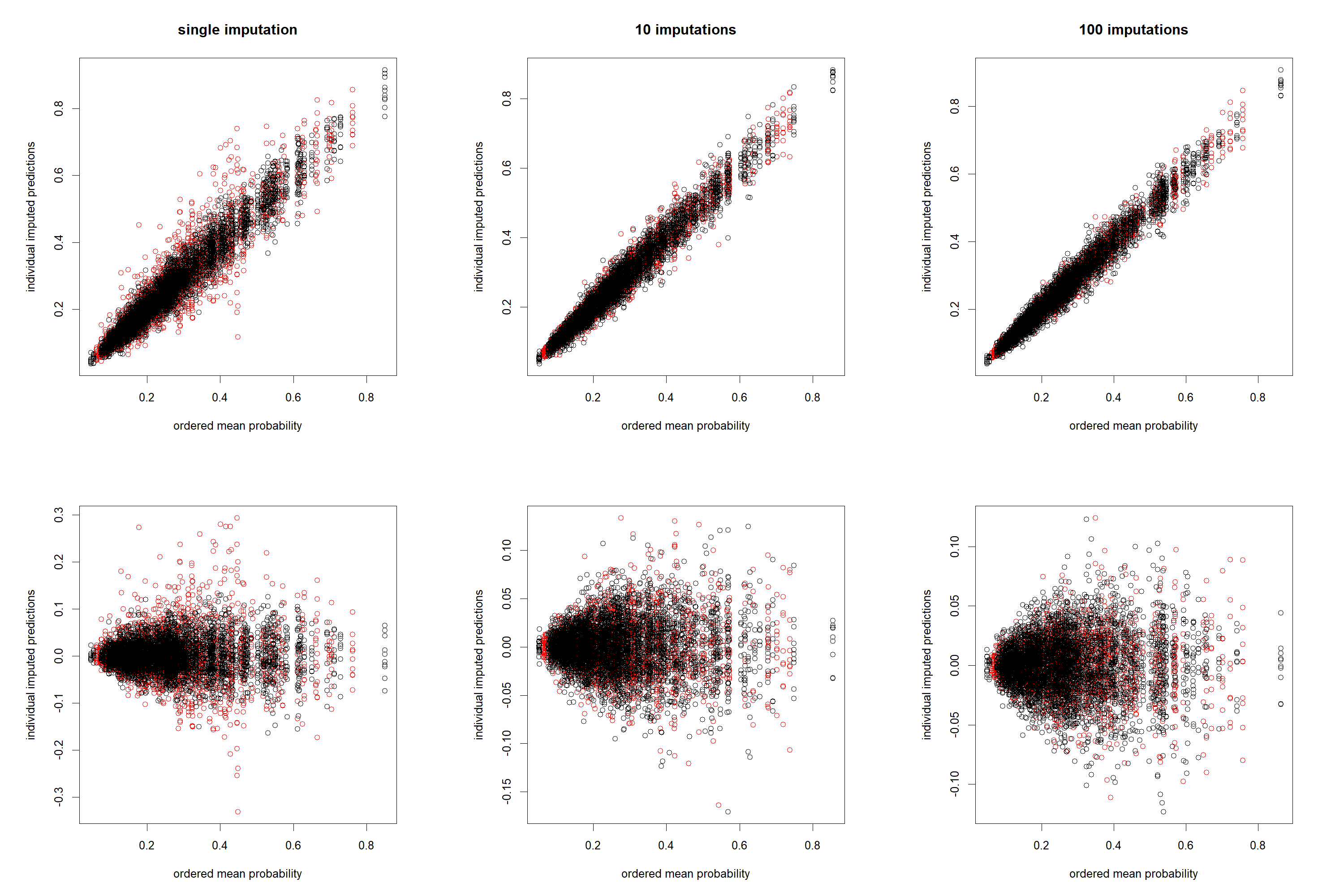We again observe prediction on partially observed records to have lower levels of (between-replication) variation as compared to prediction on fully observed data. Variation reduces substantially when the numbers of imputations used are increased. Observation with mean predicted probabilities close to either 0 or 1 have lower between-replication variation. Note however that the dispersal seem to be larger as compared to results from the averaging method and this effect is particularly noticeable for the higher numbers of imputations.

## Summarize predictive performance using variance functions

To further study the variation due to imputation, we summarize variation using the `R’ statistics as defined in the paper (ref). First define a function that calculates the required statistics on the replicated analyses.

``````R.statistic <- function(reps, miss){
resmat<-matrix(NA,nrow=2,ncol=7)
dimnames(resmat)<-list(c("missing","observed"),
c("R (range)","q10","median","q90","missing","replicates", "mean"))
means <- apply(reps, 1, mean)
diffs<-reps-means%*%matrix(1,nrow=1,ncol=ncol(reps))  # remove means

# variation between p=0.2 and 0.8,  and fully observed records
diffssel<-diffs[means<0.8&means>0.2&miss==0,] # select between 0.2 and 0.8 and observed records
quant<-quantile(as.vector(diffssel),c(.10, 0.5, .90))
resmat[2,2:4]<-quant
resmat[2,1]<-quant-quant # R measure
resmat[2,5]<-0  #missing record?  1=yes, 0=no
resmat[2,6]<-ncol(reps)  # number of replicates
resmat[2,7]<-mean(as.vector(diffssel)) # mean

# variation between p=0.2 and 0.8,  and missing records
diffssel<-diffs[means<0.8&means>0.2&miss==1,] # select between 0.2 and 0.8 and missing records
quant<-quantile(as.vector(diffssel),c(.10, 0.5, .90))
resmat[1,2:4]<-quant
resmat[1,1]<-quant-quant # R measure
resmat[1,5]<-1  #missing record?  1=yes, 0=no
resmat[1,6]<-ncol(reps)  # number of replicates
resmat[1,7]<-mean(as.vector(diffssel))  # mean
resmat
}``````

Now apply the function to the replicated results from the averaging approach and combine the summary measures in a matrix. Multiply all statistics by a factor 100 so they can be read at percentage scale.

``````R1.av <- R.statistic(apply(repslist_av[],c(1,3),mean),miss)
R2.av <- R.statistic(apply(repslist_av[],c(1,3),mean),miss)
R3.av <- R.statistic(apply(repslist_av[],c(1,3),mean),miss)
# Make percentages
Rstat.av <-
rbind(R1.av, R2.av, R3.av)*matrix(rep(c(100,100,100,100,1,1,100),6),byrow=T,ncol=7)
Rstat.av.miss <- t(Rstat.av[c(1,3,5),1])
Rstat.av.obsv <- t(Rstat.av[c(2,4,6),1])``````

Now do the same for the replicated results from the rubin approach and combine the summary measures in a matrix. Multiply all statistics by a factor 100 so they can be read at percentage scale.

``````R1.rb <- R.statistic(apply(repslist_rb[],c(1,3),mean),miss)
R2.rb <- R.statistic(apply(repslist_rb[],c(1,3),mean),miss)
R3.rb <- R.statistic(apply(repslist_rb[],c(1,3),mean),miss)
# Make percentages
Rstat.rb <-
rbind(R1.rb, R2.rb, R3.rb)*matrix(rep(c(100,100,100,100,1,1,100),6),byrow=T,ncol=7)
Rstat.rb.miss <- t(Rstat.rb[c(1,3,5),1])
Rstat.rb.obsv <- t(Rstat.rb[c(2,4,6),1])``````

Produce the variance `decay’ plots for both approaches.

``````par(mfrow=c(1,2),pty="s")
index <- c(1, 10, 100)
# first plot - averaging approach.
matplot(index,cbind(t(Rstat.av.miss), t(Rstat.av.obsv)),
type="n",log="x",pch=NULL,ylim=c(0,15),axes=F,
xlab="number of imputations",ylab="percentage deviation R")
matlines(index,cbind(t(Rstat.av.miss), t(Rstat.av.obsv)),
type="b",lty=c(1,1,2,2),col=1,log="x",pch=c(1,20))
axis(1,at=index)
axis(2)
box()
title("Averaging approach")
# second plot - rubin approach.
matplot(index,cbind(t(Rstat.rb.miss), t(Rstat.rb.obsv)),
type="n",log="x",pch=NULL,ylim=c(0,15),axes=F,
xlab="number of imputations",ylab="percentage deviation R")
matlines(index,cbind(t(Rstat.rb.miss), t(Rstat.rb.obsv)),
type="b",lty=c(1,1,2,2),col=1,log="x",pch=c(1,20))
axis(1,at=c(1,10,100))
axis(2)
box()
title("Rubin approach")``````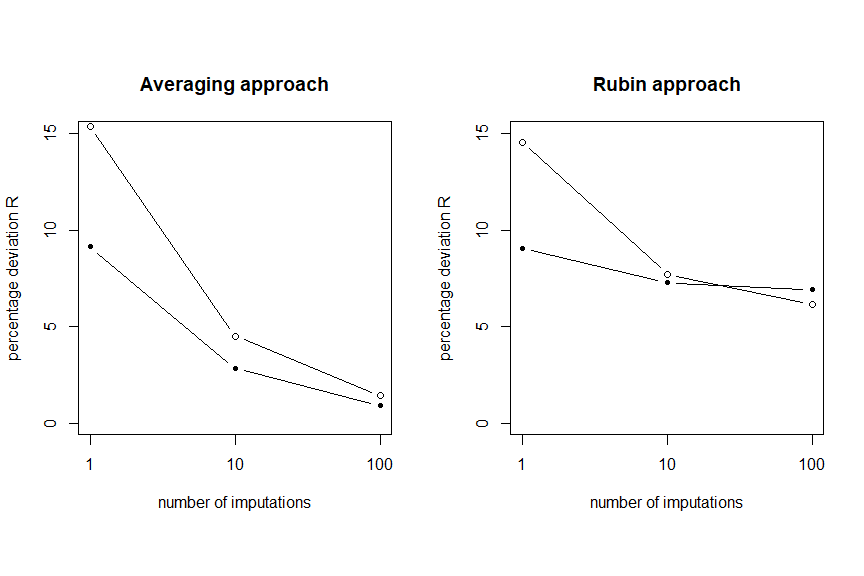Present the results as table, for approach 1 (prediction-averaging).

``````knitr::kable(round(Rstat.av, digits=2),
caption="Variance summaries of deviation relative to the mean prediction between
replicate predictions for different imputations with the averaging approach.
All statistics were multiplied by 100.")``````
Variance summaries of deviation relative to the mean prediction between replicate predictions for different imputations with the averaging approach. All statistics were multiplied by 100.
R (range) q10 median q90 missing replicates mean
missing 15.37 -7.74 -0.36 7.63 1 10 0
observed 9.13 -4.62 0.02 4.51 0 10 0
missing 4.51 -2.26 0.01 2.24 1 10 0
observed 2.84 -1.39 -0.02 1.45 0 10 0
missing 1.43 -0.72 -0.01 0.72 1 10 0
observed 0.90 -0.45 0.00 0.45 0 10 0

Present the results as table, for approach 2 (rubin).

``````knitr::kable(round(Rstat.rb, digits=2),
caption="Variance summaries of deviation relative to the mean prediction between
predictions for different imputations with the rubin approach.
All statistics were multiplied by 100.")``````
Variance summaries of deviation relative to the mean prediction between predictions for different imputations with the rubin approach. All statistics were multiplied by 100.
R (range) q10 median q90 missing replicates mean
missing 14.56 -6.82 -0.53 7.74 1 10 0
observed 9.06 -4.40 -0.04 4.66 0 10 0
missing 7.72 -3.66 -0.17 4.06 1 10 0
observed 7.27 -3.62 -0.01 3.65 0 10 0
missing 6.17 -3.16 0.05 3.02 1 10 0
observed 6.92 -3.45 -0.01 3.47 0 10 0

Next, we investigate Brier scores and AUCs. We use the `pROC` package for the AUC calculations. We again first define a function which calculates the required measures.

``````library(pROC)
#> Type 'citation("pROC")' for a citation.
#>
#> Attaching package: 'pROC'
#> The following objects are masked from 'package:stats':
#>
#>     cov, smooth, var
Briers <- function(status, reps, miss) {
resmat <- matrix(NA, nrow = 3, ncol = 4)
dimnames(resmat) <-
list(c("missing", "all", "complete"), c("Briermean", "Briersd", "AUCmean", "AUCsd"))

statustotal <- status
statusmissing <- status[miss == 1]
statusobserved <- status[miss == 0]
``````